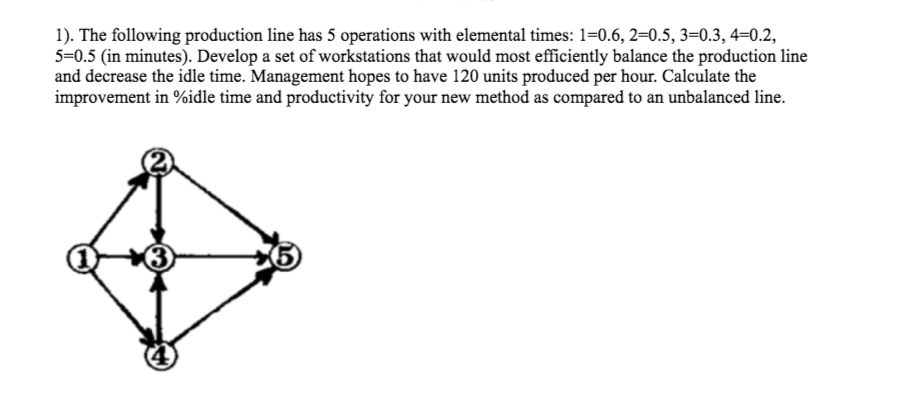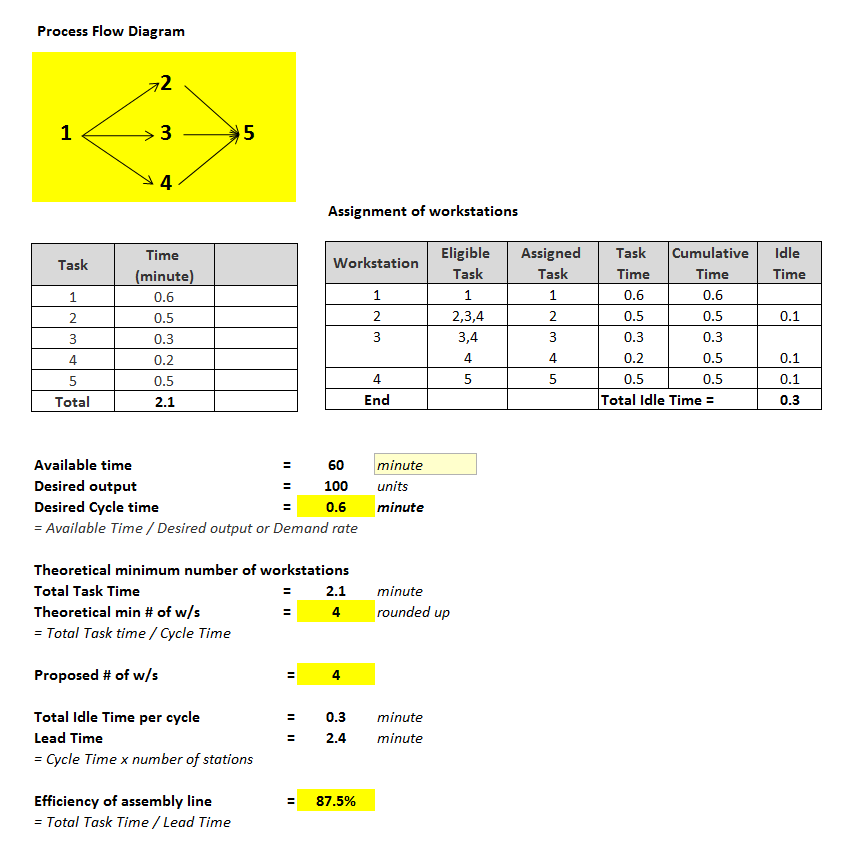# Answered! The following production line has 5 operations with elemental times: 1=0.6, 2=0.5, 3=0.3, 4=0.2, 5=0.5 (in…The following production line has 5 operations with elemental times: 1=0.6, 2=0.5, 3=0.3, 4=0.2, 5=0.5 (in minutes). Develop a set of workstations that would most efficiently balance the production line and decrease the idle time. Management hopes to have 120 units produced per hour. Calculate the improvement in %idle time and productivity for your new method as compared to an unbalanced line.

Desired output =120 units per hour

Therefore desired cycle time = 60 minutes / 120 = 0.5 minutes per unit

Don't use plagiarized sources. Get Your Custom Essay on
Answered! The following production line has 5 operations with elemental times: 1=0.6, 2=0.5, 3=0.3, 4=0.2, 5=0.5 (in…
GET AN ESSAY WRITTEN FOR YOU FROM AS LOW AS \$13/PAGE

Production line has 5 stations. Elemental time of station 1 is 0.6 minutes, which is more than the desired cycle time of 0.5 minutes. Therefore it is not possible to achieve the target production rate of 120 units per hour. The maximum possible production rate = 60/0.6 = 100 units per hour.

Assembly line balancing for the target output rate of 100 units per hour is as follows